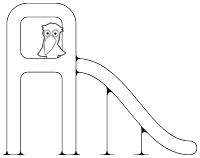### Dinfio Playground

Let's play with Dinfio!

###### Graph Example

This is a graph example using adjacency list. In this example, there are 3 classes: `graph`, `vertex`, and `edge`.
Author: Matt Hofmann

``````' Graph Example using Adjacency List

start
var graph: g = graph()

g.add_vertex("A")
g.add_vertex("B")
g.add_vertex("C")
g.add_vertex("D")

g.connect("A", "B", 2, true)   ' Connect A <-> B with cost = 2
g.connect("A", "C", 10, true)  ' Connect A <-> C with cost = 10
g.connect("A", "D", 12, false) ' Connect A  -> D with cost = 12
g.connect("C", "D", 7, false)  ' Connect C  -> D with cost = 7

g.print()
stop

class vertex
field name
field edge: edges

function construct(name)
this.name = name
this.edges = array()
stop

function add_adjacent(vertex: a, cost, undirected)
this.edges[size(this.edges)] = edge(a, cost)

if undirected
a.edges[size(a.edges)] = edge(this, cost)
endif
stop
endclass

class edge
field cost
field vertex: adjacent

function construct(vertex: a, cost)
this.cost = cost
this.adjacent = a
stop
endclass

class graph
field vertex: vertices

function construct()
this.vertices = array()
stop

function add_vertex(name)
this.vertices[size(this.vertices)] = vertex(name)
stop

function connect(vertex_1, vertex_2, cost, undirected)
var vertex: v1 = this.get_vertex(vertex_1)
var vertex: v2 = this.get_vertex(vertex_2)

if !is_nothing(v1) && !is_nothing(v2)
v1.add_adjacent(v2, cost, undirected)
endif
stop

function print()
writeln("         connected to")
writeln("Vertex ----------------> Adjacent vertices (Cost)")

for i, 0, size(this.vertices) - 1
write(this.vertices[i].name & " -> ")

for j, 0, size(this.vertices[i].edges) - 1
write(this.vertices[i].edges[j].adjacent.name & "(" & this.vertices[i].edges[j].cost & ") ")
endfor

writeln()
endfor
stop

function get_vertex(name)
yield nothing

for i, 0, size(this.vertices) - 1
if this.vertices[i].name == name
return this.vertices[i]
endif
endfor
stop
endclass``````

← Back to the Dinfio Playground / Download this program